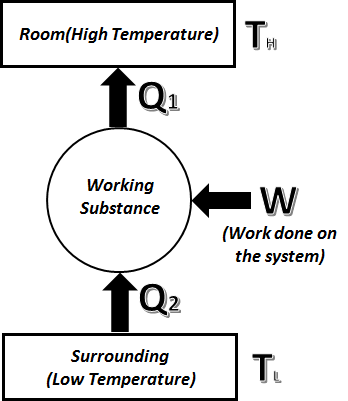# COP(Heat Pump)=COP(Refrigerator)+1

##### Heat PUmp:

Heat pump is a device that transfers heat from a colder area to a hotter area by using mechanical energy, as in a refrigerator. In heat pump how much heat is rejected at higher temperature is an important quantity.Coefficient of performance of Heat Pump$\displaystyle {{(COP)}_{H}}=\frac{{{Q}_{1}}}{W}$$\displaystyle {{Q}_{1}}=W+{{Q}_{2}}$   ⇒$\displaystyle W={{Q}_{1}}-{{Q}_{2}}$$\displaystyle {{(COP)}_{H}}=\frac{{{Q}_{1}}}{{{Q}_{1}}-{{Q}_{2}}}$ ……….(1)

##### Refrigerator:

refrigerator is a device which is designed to remove heat from a space that is at lower temperature than its surroundings. In refrigerator heat extracted from lower temperature is an important quantity.Coefficient of performance of Refrigerator$\displaystyle {{(COP)}_{R}}=\frac{{{Q}_{2}}}{W}$$\displaystyle {{Q}_{1}}=W+{{Q}_{2}}$    ⇒$\displaystyle W={{Q}_{1}}-{{Q}_{2}}$$\displaystyle {{(COP)}_{R}}=\frac{{{Q}_{2}}}{{{Q}_{1}}-{{Q}_{2}}}$ ……….(2)

From [ (1) – (2) ] we get :-$\displaystyle {{(COP)}_{H}}-{{(COP)}_{R}}=\frac{{{Q}_{1}}}{{{Q}_{1}}-{{Q}_{2}}}-\frac{{{Q}_{2}}}{{{Q}_{1}}-{{Q}_{2}}}=1$

∴ (COP)H = 1 + (COP)R

For video lecture on the above topic click the link below:-